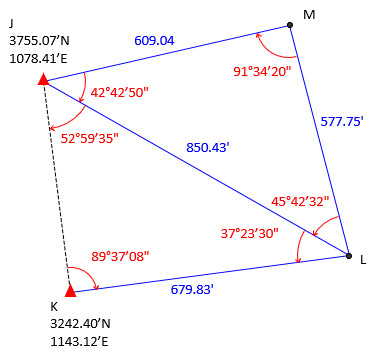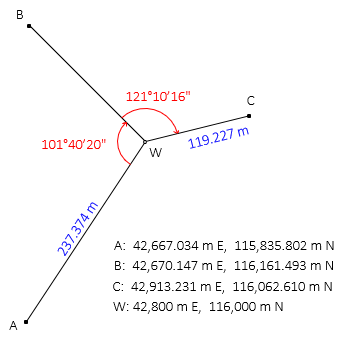### Problem (1)

Given the level circuit below, what are the adjusted elevations and their expected errors for points A and B? Compute to both to 0.001 ft.Obs dElev (ft) Len* (ft) Obs dElev (ft) Len* (ft) 1 +3.28 625 4 -2.48 1100 2 -11.81 800 5 -11.85 750 3 +14.51 550 6 +8.58 375

*Len is the sum of the backsight and foresite distances for the observation run.

Solution: Least Squares Prob 1

### Problem (2)

What are the sizes of the [C] and [K] matrices for the following horizontal network?Solution: Least Squares Prob 2

### Problem (3)

Given the horizontal network below:The initial coordinates of point W are estimated.

Create the [C] and [K] matrices.

Solution: Least Squares Prob 3

### Problem (4)

The following traverse was adjusted by least squares to determine the coordinates of points G and H.Final coordinates of G and H are:

 Point North East G 4,958.913 917.702 H 5,067.094 1,252.311

Matrices from the final iteration of the adjustment are:#### Compute:

(a) Expected uncertainties of the adjusted coordinates.